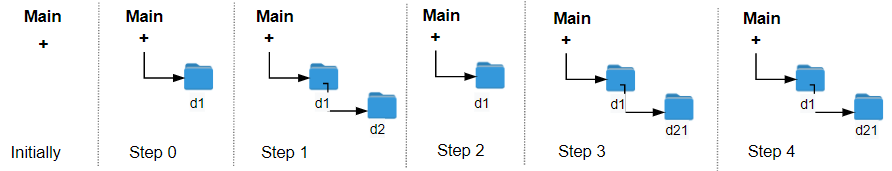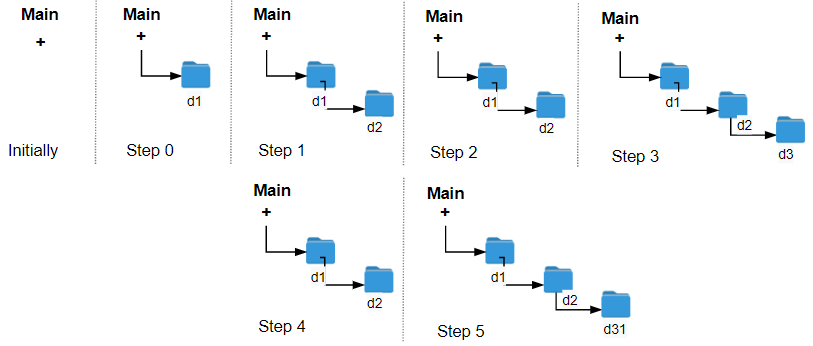# Crawler Log Folder

## 1: U-nderstand

Understand what the interviewer is asking for by using test cases and questions about the problem.

• Established a set (2-3) of test cases to verify their own solution later.
• Established a set (1-2) of edge cases to verify their solution handles complexities.
• Have fully understood the problem and have no clarifying questions.
• Have you verified any Time/Space Constraints for this problem?

Be sure that you clarify the input and output parameters of the problem:

• How should I handle an empty log file?
• All log files will have at least one input
• What are the time and space constraints?
• Time should be `O(N)` and space should be `O(1)` including the recursive stack, `N` being the length of the logs

Run through a set of example cases:

``````HAPPY CASE
Input: logs = ["d1/","d2/","../","d21/","./"]
Output: 2
Explanation: Use this change folder operation "../" 2 times and go back to the main folder.````````````Input: logs = ["d1/","d2/","./","d3/","../","d31/"]
Output: 3````````````EDGE CASE
Input: logs = ["../"]
Output: 0``````

## 2: M-atch

Match what this problem looks like to known categories of problems, e.g. Linked List or Dynamic Programming, and strategies or patterns in those categories.

• Sort
• Not helpful, we need to maintain the relative order of the logs
• Two pointer solutions (left and right pointer variables)
• Not helpful, we need to move in one direction
• Storing the elements of the array in a HashMap or a Set
• Not helpful, how does the hashmap help us find the distance between characters
• Traversing the array with a sliding window
• Not helpful, we are not looking for a window size
• Stack
• When dealing with file system traversal, stack is a good choice

## 3: P-lan

Plan the solution with appropriate visualizations and pseudocode.

General Idea: We will recursively find the depth of the file traversal logs and that will be the minimum operations to return to root.

``````1. Write a recursive function to drill into the file system.
a. Set the basecase: Out of bound.
b. Update depth base on log function string
c. Call recursive function on next log item
2. Call the recursive function
3. Return the result.``````

General Idea: We will iteratively find the depth of the file traversal logs and that will be the minimum operations to return to root.

``````1. Create a stack
3. Return the size of stack.``````

⚠️ Common Mistakes

• Using iterative approach will work here, however the interviewer wants the recursive solution.

## 4: I-mplement

Implement the code to solve the algorithm.

Recursive

``````class Solution:
def minOperations(self, logs: List[str]) -> int:
depth = 0
# Write a recursive function to drill into the file system
def helper(i):
# Set the basecase: Out of bound
if i > len(logs) - 1:
return

# Update depth base on log function string
nonlocal depth
if logs[i] == "../":
if depth > 0:
depth -= 1
elif logs[i] == "./":
pass
else:
depth  += 1

# Call recursive function on next log item
helper(i + 1)

# Call the recursive function
helper(0)

# Return the result
return depth``````
``````class Solution {
int depth = 0;
public int minOperations(String[] logs) {
// Call the recursive function
helper(logs, 0);
// Return the result
return depth;
}
// Write a recursive function to drill into the file system
private void helper(String[] logs, int i) {
// Set the basecase: Out of bound
if (i > logs.length - 1) return;

// Update depth base on log function string
if (logs[i].equals("../")) {
if (depth > 0) {
depth--;
}
} else if (logs[i].equals("./")) {
;
} else {
depth++;
}

// Call recursive function on next log item
helper(logs, i+1);
}
}``````

Iterative

``````class Solution:
def minOperations(self, logs: List[str]) -> int:
# Create a stack
stack = []

if log == "../":
if stack:
stack.pop()
elif log == "./":
continue
else:
stack.append(log)

# Return the size of stack
return len(stack)``````
``````class Solution {
public int minOperations(String[] logs) {
// Create a stack
var stack = new Stack<String>();
for(var log : logs){
if(log.equals("../")){
if(!stack.empty())
stack.pop();
}else if(log.equals("./")){

}else{
stack.push(log);
}
}
// Return the size of stack.
return stack.size();
}
}``````

## 5: R-eview

Review the code by running specific example(s) and recording values (watchlist) of your code's variables along the way.

• Trace through your code with an input to check for the expected output
• Catch possible edge cases and off-by-one errors

## 6: E-valuate

Evaluate the performance of your algorithm and state any strong/weak or future potential work.

Assume `N` represents the size of array

• Time Complexity: O(N) because we will run the algorithm once to process all logged commands
• Space Complexity: O(1) because the recusive call does not need to be stored in memory. Technically this called Tail recursion, a recursion of a function where it does not consumes stack space and hence prevents stack overflow.